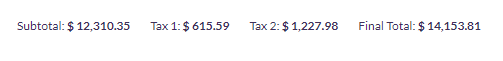#### Categories

By: Eduardo Ponce de Leon
2020-05-23

# How to add Total Sums from columns in Table Views

By: Eduardo Ponce de Leon
2020-05-23

How to add the numeric values of an entire column in a table view and show the sum using \$viewtotals

In all the table views we have the option of displaying the sum of a column on the top of a tableThis sum is only for the visible results, which means that if we are using pagination and there is more than one page, the sum is only for the current page.

For this we have one multidimensional array \$viewtotals with name, col, and prefix, for example:

```\$viewtotals['name'] = 'Subtotal';
\$viewtotals['name'] = 'Tax 1';
\$viewtotals['name'] = 'Tax 2';
\$viewtotals['name'] = 'Final Total';

\$viewtotals['col'] = 'subtotal';
\$viewtotals['col'] = 'tax2';
\$viewtotals['col'] = 'tax';
\$viewtotals['col'] = 'total';

\$viewtotals['prefix'] = '\$';
\$viewtotals['prefix'] = '\$';
\$viewtotals['prefix'] = '\$';
\$viewtotals['prefix'] = '\$';```

in col we put the name of the column of the database. The rest is just formatting